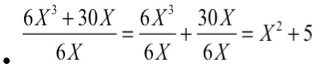Simplifying Expressions

A monomial is an algebraic expression that consists of only one term,
for example:

3a, 4f, 5x.....

A polynomial consists of two or more terms:
for example:

4x + 7y

Adding and Subtracting Polynomials:

When adding/subtracting polynomials, you need to group similar terms.

For example:

3a³-6a²+ 5a³+12a²=  8a³+6a²

12X² + X² =    13X²

4Y - 5Y= -1Y

17X² + 2X + 8X²+ 5X = 25X²+ 7X

Multiplying/Dividing Polynomials:

When multiplying or dividing polynomials, you need to use the rules of exponents.

For Example:

3X(X³+ 4X²) = 3X⁴+ 12X³

4Y⁴(Y²+ 6Y)= 4Y⁶+ 24Y⁵